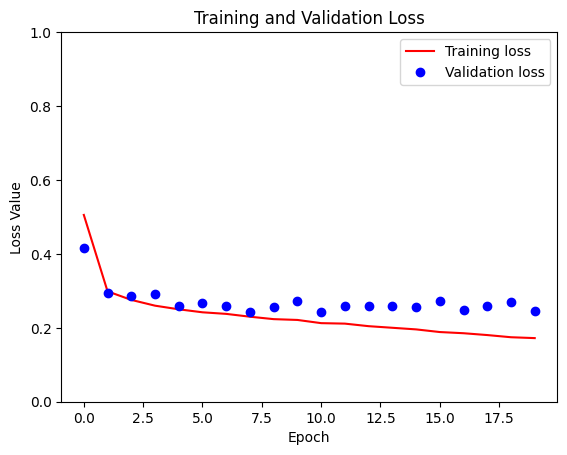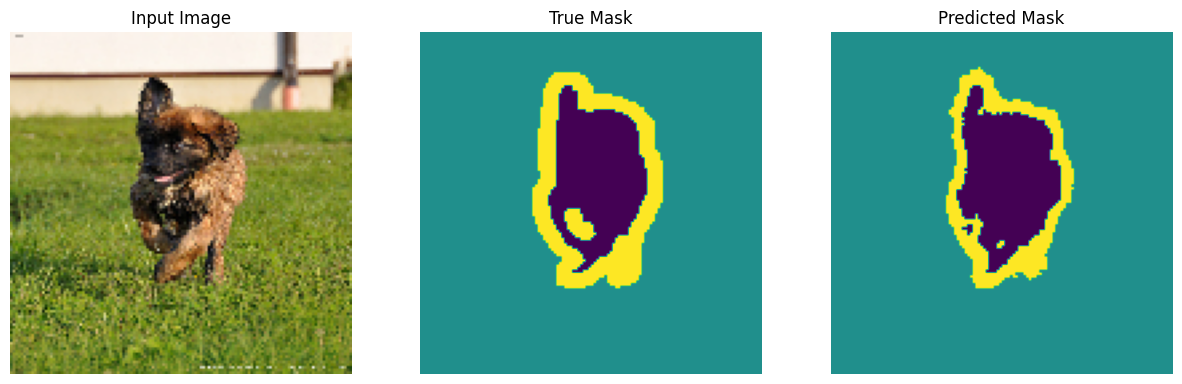TensorFlow 2.0 RC is available

# Image segmentation

This tutorial focuses on the task of image segmentation, using a modified U-Net.

## What is image segmentation?

So far you have seen image classification, where the task of the network is to assign a label or class to an input image. However, suppose you want to know where an object is located in the image, the shape of that object, which pixel belongs to which object, etc. In this case you will want to segment the image, i.e., each pixel of the image is given a label. Thus, the task of image segmentation is to train a neural network to output a pixel-wise mask of the image. This helps in understanding the image at a much lower level, i.e., the pixel level. Image segmentation has many applications in medical imaging, self-driving cars and satellite imaging to name a few.

The dataset that will be used for this tutorial is the Oxford-IIIT Pet Dataset, created by Parkhi et al. The dataset consists of images, their corresponding labels, and pixel-wise masks. The masks are basically labels for each pixel. Each pixel is given one of three categories :

• Class 1 : Pixel belonging to the pet.
• Class 2 : Pixel bordering the pet.
• Class 3 : None of the above/ Surrounding pixel.
``````!pip install -q git+https://github.com/tensorflow/examples.git
``````
``````try:
# %tensorflow_version only exists in Colab.
%tensorflow_version 2.x
except Exception:
pass
import tensorflow as tf
``````
``````from __future__ import absolute_import, division, print_function, unicode_literals

from tensorflow_examples.models.pix2pix import pix2pix

import tensorflow_datasets as tfds
tfds.disable_progress_bar()

from IPython.display import clear_output
import matplotlib.pyplot as plt
``````

## Download the Oxford-IIIT Pets dataset

The dataset is already included in TensorFlow datasets, all that is needed to do is download it. The segmentation masks are included in version 3.0.0, which is why this particular version is used.

``````dataset, info = tfds.load('oxford_iiit_pet:3.0.0', with_info=True)
``````
```Downloading and preparing dataset oxford_iiit_pet (801.24 MiB) to /home/kbuilder/tensorflow_datasets/oxford_iiit_pet/3.0.0...
Shuffling and writing examples to /home/kbuilder/tensorflow_datasets/oxford_iiit_pet/3.0.0.incomplete3V08VG/oxford_iiit_pet-train.tfrecord
Shuffling and writing examples to /home/kbuilder/tensorflow_datasets/oxford_iiit_pet/3.0.0.incomplete3V08VG/oxford_iiit_pet-test.tfrecord

WARNING: Logging before flag parsing goes to stderr.
W0813 05:18:23.102592 140228796684032 dataset_builder.py:439] Warning: Setting shuffle_files=True because split=TRAIN and shuffle_files=None. This behavior will be deprecated on 2019-08-06, at which point shuffle_files=False will be the default for all splits.
W0813 05:18:36.589790 140228796684032 dataset_builder.py:439] Warning: Setting shuffle_files=True because split=TRAIN and shuffle_files=None. This behavior will be deprecated on 2019-08-06, at which point shuffle_files=False will be the default for all splits.

Dataset oxford_iiit_pet downloaded and prepared to /home/kbuilder/tensorflow_datasets/oxford_iiit_pet/3.0.0. Subsequent calls will reuse this data.
```

The following code performs a simple augmentation of flipping an image. In addition, image is normalized to [0,1]. Finally, as mentioned above the pixels in the segmentation mask are labeled either {1, 2, 3}. For the sake of convinience, let's subtract 1 from the segmentation mask, resulting in labels that are : {0, 1, 2}.

``````def normalize(input_image, input_mask):
input_image = tf.cast(input_image, tf.float32)/128.0 - 1
input_mask -= 1
return input_image, input_mask
``````
``````@tf.function
def load_image_train(datapoint):
input_image = tf.image.resize(datapoint['image'], (128, 128))
input_mask = tf.image.resize(datapoint['segmentation_mask'], (128, 128))

if tf.random.uniform(()) > 0.5:
input_image = tf.image.flip_left_right(input_image)
input_mask = tf.image.flip_left_right(input_mask)

input_image, input_mask = normalize(input_image, input_mask)

return input_image, input_mask
``````
``````def load_image_test(datapoint):
input_image = tf.image.resize(datapoint['image'], (128, 128))
input_mask = tf.image.resize(datapoint['segmentation_mask'], (128, 128))

input_image, input_mask = normalize(input_image, input_mask)

return input_image, input_mask
``````

The dataset already contains the required splits of test and train and so let's continue to use the same split.

``````TRAIN_LENGTH = info.splits['train'].num_examples
BATCH_SIZE = 64
BUFFER_SIZE = 1000
STEPS_PER_EPOCH = TRAIN_LENGTH // BATCH_SIZE
``````
``````train = dataset['train'].map(load_image_train, num_parallel_calls=tf.data.experimental.AUTOTUNE)
test = dataset['test'].map(load_image_test)
``````
``````train_dataset = train.cache().shuffle(BUFFER_SIZE).batch(BATCH_SIZE).repeat()
train_dataset = train_dataset.prefetch(buffer_size=tf.data.experimental.AUTOTUNE)
test_dataset = test.batch(BATCH_SIZE)
``````

Let's take a look at an image example and it's correponding mask from the dataset.

``````def display(display_list):
plt.figure(figsize=(15, 15))

title = ['Input Image', 'True Mask', 'Predicted Mask']

for i in range(len(display_list)):
plt.subplot(1, len(display_list), i+1)
plt.title(title[i])
plt.imshow(tf.keras.preprocessing.image.array_to_img(display_list[i]))
plt.axis('off')
plt.show()
``````
``````for image, mask in train.take(1):
sample_image, sample_mask = image, mask
display([sample_image, sample_mask])
``````## Define the model

The model being used here is a modified U-Net. A U-Net consists of an encoder (downsampler) and decoder (upsampler). In-order to learn robust features, and reduce the number of trainable parameters, a pretrained model can be used as the encoder. Thus, the encoder for this task will be a pretrained MobileNetV2 model, whose intermediate outputs will be used, and the decoder will be the upsample block already implemented in TensorFlow Examples in the Pix2pix tutorial.

The reason to output three channels is because there are three possible labels for each pixel. Think of this as multi-classification where each pixel is being classified into three classes.

``````OUTPUT_CHANNELS = 3
``````

As mentioned, the encoder will be a pretrained MobileNetV2 model which is prepared and ready to use in tf.keras.applications. The encoder consists of specific outputs from intermediate layers in the model. Note that the encoder will not be trained during the training process.

``````base_model = tf.keras.applications.MobileNetV2(input_shape=[128, 128, 3], include_top=False)

# Use the activations of these layers
layer_names = [
'block_1_expand_relu',   # 64x64
'block_3_expand_relu',   # 32x32
'block_6_expand_relu',   # 16x16
'block_13_expand_relu',  # 8x8
'block_16_project',      # 4x4
]
layers = [base_model.get_layer(name).output for name in layer_names]

# Create the feature extraction model
down_stack = tf.keras.Model(inputs=base_model.input, outputs=layers)

down_stack.trainable = False
``````
```Downloading data from https://github.com/JonathanCMitchell/mobilenet_v2_keras/releases/download/v1.1/mobilenet_v2_weights_tf_dim_ordering_tf_kernels_1.0_128_no_top.h5
9412608/9406464 [==============================] - 2s 0us/step
```

The decoder/upsampler is simply a series of upsample blocks implemented in TensorFlow examples.

``````up_stack = [
pix2pix.upsample(512, 3),  # 4x4 -> 8x8
pix2pix.upsample(256, 3),  # 8x8 -> 16x16
pix2pix.upsample(128, 3),  # 16x16 -> 32x32
pix2pix.upsample(64, 3),   # 32x32 -> 64x64
]
``````
``````def unet_model(output_channels):

# This is the last layer of the model
last = tf.keras.layers.Conv2DTranspose(
output_channels, 3, strides=2,
padding='same', activation='softmax')  #64x64 -> 128x128

inputs = tf.keras.layers.Input(shape=[128, 128, 3])
x = inputs

# Downsampling through the model
skips = down_stack(x)
x = skips[-1]
skips = reversed(skips[:-1])

# Upsampling and establishing the skip connections
for up, skip in zip(up_stack, skips):
x = up(x)
concat = tf.keras.layers.Concatenate()
x = concat([x, skip])

x = last(x)

return tf.keras.Model(inputs=inputs, outputs=x)
``````

## Train the model

Now, all that is left to do is to compile and train the model. The loss being used here is losses.sparse_categorical_crossentropy. The reason to use this loss function is because the network is trying to assign each pixel a label, just like multi-class prediction. In the true segmentation mask, each pixel has either a {0,1,2}. The network here is outputting three channels. Essentially, each channel is trying to learn to predict a class, and losses.sparse_categorical_crossentropy is the recommended loss for such a scenario. Using the output of the network, the label assigned to the pixel is the channel with the highest value. This is what the create_mask function is doing.

``````model = unet_model(OUTPUT_CHANNELS)
model.compile(optimizer='adam', loss='sparse_categorical_crossentropy',
metrics=['accuracy'])
``````

Let's try out the model to see what it predicts before training.

``````def create_mask(pred_mask):
pred_mask = tf.argmax(pred_mask, axis=-1)
pred_mask = pred_mask[..., tf.newaxis]
return pred_mask
``````
``````def show_predictions(dataset=None, num=1):
if dataset:
for image, mask in dataset.take(num):
pred_mask = model.predict(image)
display([image, mask, create_mask(pred_mask)])
else:
display([sample_image, sample_mask,
create_mask(model.predict(sample_image[tf.newaxis, ...]))])
``````
``````show_predictions()
``````Let's observe how the model improves while it is training. To accomplish this task, a callback function is defined below.

``````class DisplayCallback(tf.keras.callbacks.Callback):
def on_epoch_end(self, epoch, logs=None):
clear_output(wait=True)
show_predictions()
print ('\nSample Prediction after epoch {}\n'.format(epoch+1))
``````
``````EPOCHS = 20
VAL_SUBSPLITS = 5
VALIDATION_STEPS = info.splits['test'].num_examples//BATCH_SIZE//VAL_SUBSPLITS

model_history = model.fit(train_dataset, epochs=EPOCHS,
steps_per_epoch=STEPS_PER_EPOCH,
validation_steps=VALIDATION_STEPS,
validation_data=test_dataset,
callbacks=[DisplayCallback()])
`````````
Sample Prediction after epoch 20

57/57 [==============================] - 4s 73ms/step - loss: 0.1445 - accuracy: 0.9344 - val_loss: 0.3345 - val_accuracy: 0.8859
```
``````loss = model_history.history['loss']
val_loss = model_history.history['val_loss']

epochs = range(EPOCHS)

plt.figure()
plt.plot(epochs, loss, 'r', label='Training loss')
plt.plot(epochs, val_loss, 'bo', label='Validation loss')
plt.title('Training and Validation Loss')
plt.xlabel('Epoch')
plt.ylabel('Loss Value')
plt.ylim([0, 1])
plt.legend()
plt.show()
``````## Make predictions

Let's make some predictions. In the interest of saving time, the number of epochs was kept small, but you may set this higher to achieve more accurate results.

``````show_predictions(test_dataset, 3)
``````## Next steps

Now that you have an understanding of what image segmentation is and how it works, you can try this tutorial out with different intermediate layer outputs, or even different pretrained model. You may also challenge yourself by trying out the Carvana image masking challenge hosted on Kaggle.

You may also want to see the Tensorflow Object Detection API for another model you can retrain on your own data.